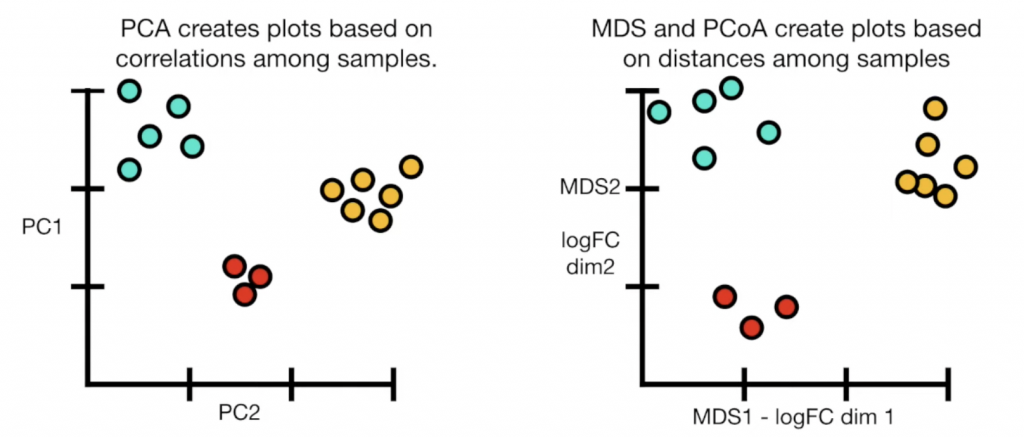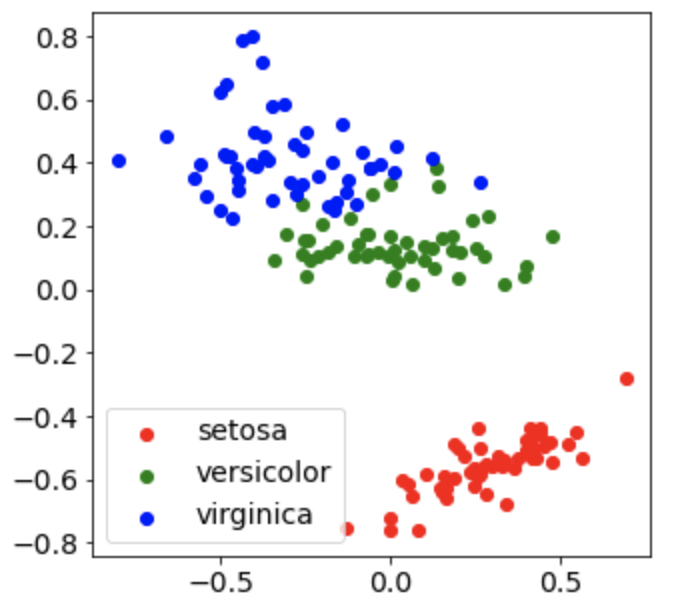#DAY 8
0
AI & Data

## MDS原理

MDS主要的原則是保持“距離”不變，但這裡的距離是可以變換的，我們可以使用最簡單的euclidean distance，也可以使用第一天提到的DTW，要注意的是如果使用euclidean distance，跑出的分佈結果會和PCA相同。另外一個要注意的地方是因為牽涉到距離，所以要執行MDS的資料必須要先標準化（normalization）。## Python

``````import numpy as np
import matplotlib.pyplot as plt
from sklearn.manifold import MDS
from sklearn.preprocessing import MinMaxScaler

X = data.data

scaler = MinMaxScaler()
X_scaled = scaler.fit_transform(X)
``````

MDS的重要參數有：

1. n_components：想要降低成幾維度的資料
2. dissimilarity（default=’euclidean’）：若要放入別的distance metric則輸入‘precomputed’，並在fit_transform語法中放入metric
``````mds = MDS(n_components=2,random_state=0)
X_2d = mds.fit_transform(X_scaled)
``````
``````colors = ['red','green','blue']

for i in np.unique(data.target):
subset = X_2d[data.target == i]
x = [row for row in subset]
y = [row for row in subset]
plt.scatter(x,y,c=colors[i],label=data.target_names[i])
plt.legend()
plt.show()
``````